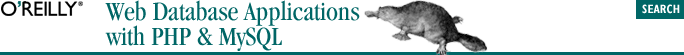home | O'Reilly's CD bookshelfs | FreeBSD | Linux | Cisco | Cisco Exam## 3.9. Functions

### 3.9.1. Arithmetic and comparison operators

#### Table 3-2. Using the arithmetic and comparison operators

Statement

Output

`SELECT 8+3*2;`
`14`
`SELECT (8+3)*2;`
`22`
`SELECT 2=2;`
`1`
`SELECT 1!=2;`
`1`
`SELECT 2<=2;`
`1`
`SELECT 3<=2;`
`0`

The comparison operators include =, !=, <, >, <=, and >=. Four examples are shown in Table 3-2. If an expression evaluates as true, the output is 1; if an expression evaluates as false, the output is 0. To test for equality, a single equals sign is used; this contrasts with PHP, where the double equals (==) is used for equality tests, and a single equals sign is used for assignment.

To test whether two items are equal, the != operator is provided. Less-than-or-equal-to is represented by <=, and greater-than-or-equal-to is represented by >=. Parentheses can explicitly express the evaluation order.

### 3.9.1.2. Mathematical functions

We make little use of the mathematical functions provided by MySQL in this book. However, Table 3-4 shows selected MySQL mathematical functions and their output.

#### Table 3-4. Using the MySQL mathematical functions

Statement

Output

`SELECT abs(-33);`
`33`
`SELECT abs(33);`
`33`
`SELECT mod(10,3);`
`1`
`SELECT 10 % 3;`
`1`
`SELECT floor(3.14159);`
`3`
`SELECT ceiling(3.14159);`
`4`
`SELECT round(3.14159);`
`3`
`SELECT log(100);`
`4.605170`
`SELECT log10(100);`
`2`
`SELECT pow(2,3);`
`8`
`SELECT sqrt(36);`
`6`
`SELECT sin(pi( ));`
`0.000000`
`SELECT cos(pi( ));`
`-1.000000`
`SELECT tan(pi( ));`
`-0.000000`
`SELECT rand( );`
`0.8536`
`SELECT truncate(3.14159,3);`
`3.141`
`SELECT format(12345.23,0);`
`12,345`
`SELECT format(12345.23, 1);`
`12,345.2`

Several of the functions in Table 3-4 require some explanation:

• The abs( ) operator returns the absolute value of a number; that is, it removes the negative sign from negative numbers.

• The modulo operator—which has two identical variants, % and mod( )—divides the first number by the second number and outputs the remainder.

• The floor( ) and ceiling( ) functions are complementary: floor( ) returns the largest integer not greater than the parameter; ceiling( ) returns the smallest integer not less than the parameter.

• The round( ) function rounds to the nearest integer.

• Both the natural logarithm, log( ), and base-10 logarithm, log10( ), are available.

• The pow( ) function raises the first number to the power of the second.

• sqrt( ) takes the square root of the parameter.

• The trigonometry functions sin( ), cos( ), and tan( ) take values expressed in radians as parameters. The complementary arc sin, arc cos, and arc tan are available as asin( ), acos( ), and atan( ).

• The rand( ) function returns a pseudorandom number in the range 0 to 1.

• The truncate( ) function removes decimal places without rounding.

• The format( ) function isn't really a mathematical function but is instead used for returning numbers in a predefined format. The first parameter is the number, and the second parameter is the number of decimal places to return. The first parameter is rounded so that, for example, 123.56 formatted to one decimal place is 123.6. This function is seldom used in web database applications, because formatting is usually performed in PHP scripts.